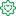Announcements
Product Release Webinar: Qlik Insider airing December 6! REGISTER TODAY!
cancel
Showing results for
Did you mean:Former Employee

## How to Build the Kaplan-Meier Curve

I had a request to build a Kaplan-Meier Curve recently and was supplied the following link which explains how the curve can be calculated: Using the sample data supplied in the example I prepared the attached application as a proposed solution. (In case the link gets taken down I have attached the pdf that it points to).

The sample data provides survival periods for ten subjects, listed below in ascending order they are: 2, 15+, 17, 18, 18+, 20+, 23, 25+, 30+, 31. (The ‘+’ sign signifies that the patient was alive at the end of the study and after any follow-up). Note that the calculations are done for days where subjects actually died - at other days results are ‘censored’ and the survival calculations do not change.

It took me a while to work out what censored actually meant. Looking at the calculations it seems the censored members are removed from the set of individuals are risk (assumed cured?). This has an indirect impact on the calculations as the Kaplan Meier number is essentially Deaths / Individuals At Risk.

Rather than try to build up one big complex formula, I built up the calculation progressively with multiple expressions that are dependant on each other. Then the final expression is the only one that is charted. This makes complex calculations like this much more manageable.

I hope you find this useful.